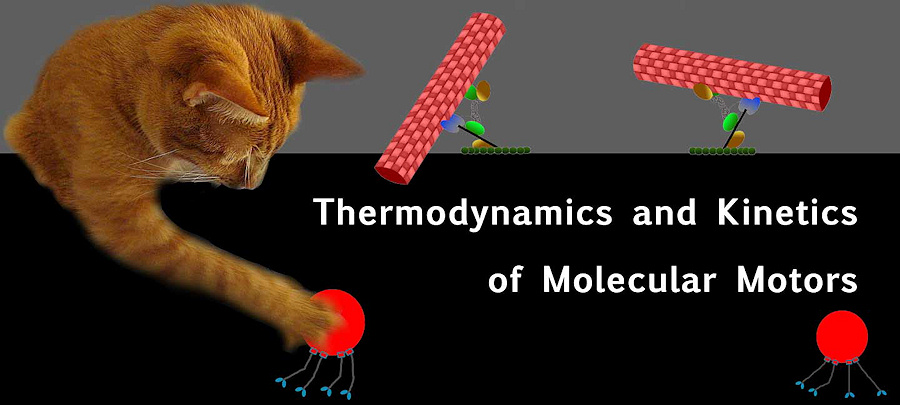# Molecular Motorsamazing picture. (source)

All motors rectify thermal motion. To make a molecular motor its potential energy $U(x)$ needs to have one of the two following properties.

1. $U(x)$ is modulated in time. That is to say the potential energy is somehow turned on and off.
2. $U(x)$ is modulated in space. The potential energy is repeatedly altered through space.

The first scenario is how one-headed motors work. The second scenario is how two-headed motors work.

## Single Headed Motors

Two ingredients go into making a single headed motor:

1. Asymmetric potential in space.
2. Potential that switches on and off with time.

### Speed and Efficiency

Assume that the potential has an asymmetric saw-tooth shape. The length of one saw-tooth is $d$ and the length of the steeper half of the saw-tooth is $\alpha d$. Let the maximum potential be $U_\text{max}=\mathcal{E}$, and let $% $. After a time $\Delta t$ the potential will turn off.

• Initially motor is in a potential minimum.
• After the potential is turned off the motor can freely diffuse for a time $\Delta t$ until the potential turns back on.

We know that the position probability distribution for 1D diffusion will be Gaussian. To make things simple on ourselves lets assume that the distribution is triangular.

Note: $P(x)$ must be wide enough to go into both neighboring saw-tooth minimums to get any useful results.

The width $w$ is a parameter set by the motor while $\alpha$ and $d$ are set by the actin filament the motor crawls on.

We can calculate the variance in position.

Lets calculate the probability that the motor will move to the notch on the right.

The probability to move to the left will then be…

The probability that the motor makes net forward motion is then the difference between these two probabilities.

Let’s check some limits to make sure that this answer makes sense.

#### Optimal $w$

For simplicity let $y\equiv\frac{d}{w}$. In order to find the optimal width we will take the derivative of the net probability with respect to $w$.

This corresponds to a maximal net probability.

A realistic value for $P_\text{net}$ for microtubules is $P_\text{net}\approx 1/10$. Using this we can calculate the net velocity of the motor.

Written with StackEdit.

Written on February 24, 2015